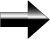Asymptotes

An asymptote is essentially a value that a particular function approaches but can never actually reach.  Before you learn the material in this section, you should be familiar with the concept of limits, as well as where certain functions become undefined (such as fractions with a zero in the denominator).

There are 3 types of asymptotes:  Vertical, Horizontal, and Slant.

Vertical Asymptotes:  The equations for these will be of the form of x ≠ some constant.  These represent a situation where x cannot be a certain value.  Vertical asymptotes exist where x does not equal something.  This will usually occur in rational functions (because the denominator can’t be zero) or in functions that contain an even root (because we can’t take a square root of a negative number).

Horizontal Asymptotes:  The equation for these will be of the form y ≠ some constant.  This represents a situation where y cannot equal a certain value.  The horizontal asymptote represents the behavior of the function as x gets closer to negative and positive infinity.  Two situations will create a horizontal asymptote:

The degree of the numerator is equal to the degree of the denominator:  In this instance, we will have a horizontal asymptote.  Remember that the idea of an asymptote is closely related to the concept of a limit.

The degree of the numerator is less than the degree of the denominator:    In this case, we will have a horizontal asymptote at y = 0.  This is because as x approaches negative or positive infinity, the denominator becomes very large – so large when compared to the numerator that the value of the entire expression goes to zero.  At positive or negative infinity, the value of the function is equal to zero, and since we can never get to infinity, the function can never get to zero – hence the asymptote at y = 0.

If the degree of the numerator is one greater than the degree of the denominator, then the function will have a slant asymptote.

Slant Asymptotes:  A function will have a slant asymptote if the degree of the numerator is greater than the degree of the denominatorThis scenario leads to a slant asymptote.

The equation for this asymptote will be a diagonal line of the form y = mx + b.  This situation happens when we have a rational function whose numerator has a higher degree than its denominator.  To find the equation for the slant asymptote, we divide the numerator by the denominator using a method like polynomial or synthetic division.